JEE  >  Test: Centre Of Mass (Competition Level 1)- 2

# Test: Centre Of Mass (Competition Level 1)- 2

Test Description

## 30 Questions MCQ Test Physics For JEE | Test: Centre Of Mass (Competition Level 1)- 2

Test: Centre Of Mass (Competition Level 1)- 2 for JEE 2023 is part of Physics For JEE preparation. The Test: Centre Of Mass (Competition Level 1)- 2 questions and answers have been prepared according to the JEE exam syllabus.The Test: Centre Of Mass (Competition Level 1)- 2 MCQs are made for JEE 2023 Exam. Find important definitions, questions, notes, meanings, examples, exercises, MCQs and online tests for Test: Centre Of Mass (Competition Level 1)- 2 below.
Solutions of Test: Centre Of Mass (Competition Level 1)- 2 questions in English are available as part of our Physics For JEE for JEE & Test: Centre Of Mass (Competition Level 1)- 2 solutions in Hindi for Physics For JEE course. Download more important topics, notes, lectures and mock test series for JEE Exam by signing up for free. Attempt Test: Centre Of Mass (Competition Level 1)- 2 | 30 questions in 60 minutes | Mock test for JEE preparation | Free important questions MCQ to study Physics For JEE for JEE Exam | Download free PDF with solutions
 1 Crore+ students have signed up on EduRev. Have you?
Test: Centre Of Mass (Competition Level 1)- 2 - Question 1

### A square plate and a circular plate made up of same material are placed touching each other on a horizontal table. If the side length of square plate is equal to diameter of the circular plate, then the centre of mass of the combination will be

Detailed Solution for Test: Centre Of Mass (Competition Level 1)- 2 - Question 1
Let m be the mass per unit area.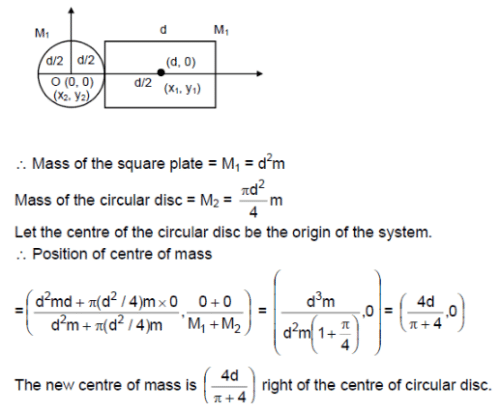Test: Centre Of Mass (Competition Level 1)- 2 - Question 2

### A rigid body consists of a 3 kg mass located at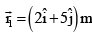and a 2 kg mass located at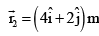.The position of center of mass is

Test: Centre Of Mass (Competition Level 1)- 2 - Question 3

### A dog weighing 5 kg is standing on a flat boat so that it is 10 meters from the shore. It walks 4 m on the boat towards the shore and then halts. The boat weighs 20 kg and one can assume that there is no friction between it and water. The dog from the shore at the end of this time is

Detailed Solution for Test: Centre Of Mass (Competition Level 1)- 2 - Question 3

Distance from shore = (10 – l + d)

Test: Centre Of Mass (Competition Level 1)- 2 - Question 4

Particles of masses 1 kg and 3 kg are at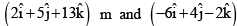m  then instantaneous position of their centre of mass is

Detailed Solution for Test: Centre Of Mass (Competition Level 1)- 2 - Question 4

xcom=m1x1+m2x2/m1+m2
=1x2+3x (-6)
=-4î
vcom= m1y1+m2y2/m1+m2
=1x5+3x4/4
=17/4 ĵ
zcom= m1z1+m2z2/m1+m2
=13-6/4
=7/4 k̂
rcom=-4î+17/4 ĵ+7/4 k̂
=1/4(-16î+17ĵ+7k̂)

Test: Centre Of Mass (Competition Level 1)- 2 - Question 5

Two bodies of masses 5 kg and 3 kg are moving towards each other with 2 m/s and 4 m/s respectively. Then velocity of centre of mass is

Detailed Solution for Test: Centre Of Mass (Competition Level 1)- 2 - Question 5

Vcm = M1V1 + M2V2/M1+M2
So 10 - 12/8 = -0.25. Now, we assumed that +x is forward (i.e. towards 3kg) but since Vcm turns out -ve it is towards 5kg block.

Test: Centre Of Mass (Competition Level 1)- 2 - Question 6

A uniform metre rod is bent into L shape with the bent arms at 90o to each other. The distance of the centre of mass from the bent point is

Detailed Solution for Test: Centre Of Mass (Competition Level 1)- 2 - Question 6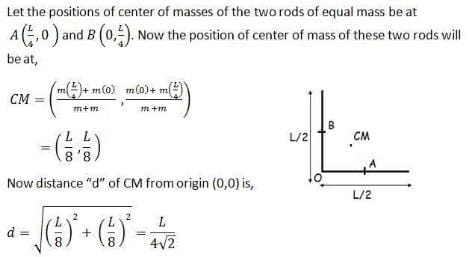Test: Centre Of Mass (Competition Level 1)- 2 - Question 7

A uniform straight rod is placed in vertical position on a smooth horizontal surface and released. As the rod is in motion, the centre of mass moves

Detailed Solution for Test: Centre Of Mass (Competition Level 1)- 2 - Question 7

If you draw the free body diagram of the rod you will find that in X direction there will be no force acting on the rod and in Y direction there is weight and normal force acting on the rod. Hence, there is no external force in X direction to its centre of mass will not move in X direction but will move only in Y direction as the forces are acting in that direction only.

Test: Centre Of Mass (Competition Level 1)- 2 - Question 8

A thin uniform rod of length L is bent at its mid point as shown in the figure. The distance of the centre of mass from the point O is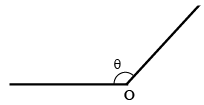Test: Centre Of Mass (Competition Level 1)- 2 - Question 9

Four bodies of masses 1, 2, 3, 4 kg respectively are placed at the corners of a square of side ‘a’. Coordinates of centre of mass are (take 1 kg at the origin, 2 kg on x-axis and 4 kg on y-axis)

Test: Centre Of Mass (Competition Level 1)- 2 - Question 10

Two identical thin uniform rods of length L each are joined to form T shape as shown in the figure. The distance of centre of mass from D is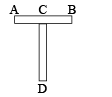Detailed Solution for Test: Centre Of Mass (Competition Level 1)- 2 - Question 10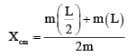On solving we get 3L/4

Test: Centre Of Mass (Competition Level 1)- 2 - Question 11

Figure shows a square plate of uniform thickness and side length √2 m. One fourth of the plate is removed as indicated. The distance of centre of mass of the remaining portion from the centre of the original square plate is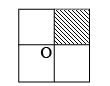Test: Centre Of Mass (Competition Level 1)- 2 - Question 12

Two particles of equal masses have velocities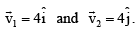First particle has an acceleration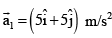while the acceleration of the other particle is zero. The centre of mass of the two particles moves in a path of

Detailed Solution for Test: Centre Of Mass (Competition Level 1)- 2 - Question 12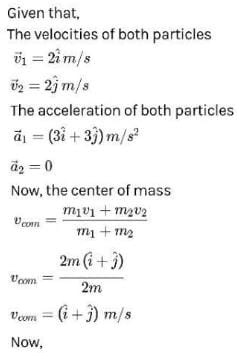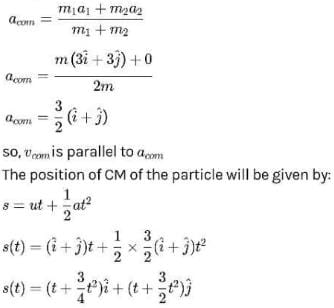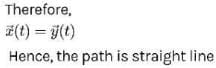Test: Centre Of Mass (Competition Level 1)- 2 - Question 13

The centre of mass of a non uniform rod of length L whose mass per unit length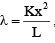where k is a constant and x is the distance from one end is

Test: Centre Of Mass (Competition Level 1)- 2 - Question 14

In the Fig. shown, pulley is tight, frictionless and string is inextensible. System is released at t = 0, at t = 2 sec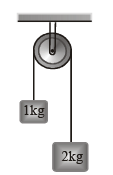Test: Centre Of Mass (Competition Level 1)- 2 - Question 15

Two bodies of masses m1 and m2 are moving with velocity v1 and v2 respectively in the same direction. The total momentum of the system in the frame of reference attached the centre of mass is (v is relative velocity between the masses)

Detailed Solution for Test: Centre Of Mass (Competition Level 1)- 2 - Question 15

P = m1(v1 - vc) + m2(v2 – vc)
P = m1v1 + m2v2 – (m1 + m2)vc
Also, we know that vc = m1v1 + m2v2/(m1 + m2)
Hence P = 0

Test: Centre Of Mass (Competition Level 1)- 2 - Question 16

A body of mass m moving at a constant velocity v hits another body of the same mass moving with a velocity v/2 but in the opposite direction and sticks to it. The common velocity after collision is

Detailed Solution for Test: Centre Of Mass (Competition Level 1)- 2 - Question 16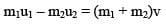Hence v = v/4

Test: Centre Of Mass (Competition Level 1)- 2 - Question 17

A rubber ball is dropped from a height of 5 m on a planet where the acceleration due to gravity is not known. On bouncing, it rises to 1.8 m. The ball loses its velocity on bouncing by a factor of

Test: Centre Of Mass (Competition Level 1)- 2 - Question 18

A body of mass 5 kg is acted on by a net force F which varies with time t as shown in graph, then the net momentum in SI units gained by the body at the end of 10 seconds is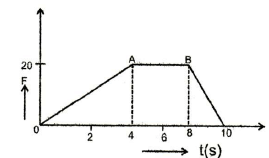Detailed Solution for Test: Centre Of Mass (Competition Level 1)- 2 - Question 18

Area under F-t curve = change in momentum.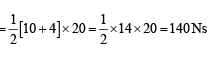Test: Centre Of Mass (Competition Level 1)- 2 - Question 19

A force time graph for the motion of a body is as shown in figure. Change in linear momentum between 0 and 6 s is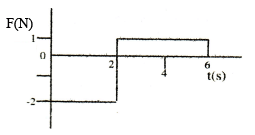Detailed Solution for Test: Centre Of Mass (Competition Level 1)- 2 - Question 19

Area under F-t curve = change in momentum = 2 x (-2) + 1 x 4 = -4 + 4 = 0

Test: Centre Of Mass (Competition Level 1)- 2 - Question 20

A body is acted on by a force given by F = (10 + 2t) N. The impulse received by the body during the first four seconds is

Test: Centre Of Mass (Competition Level 1)- 2 - Question 21

An impulse is applied to a moving object with the force at an angle of 120o with the velocity vector. The angle between the impulse vector and the change in momentum vector is

Detailed Solution for Test: Centre Of Mass (Competition Level 1)- 2 - Question 21

Conceptual

Test: Centre Of Mass (Competition Level 1)- 2 - Question 22

A ball of mass 10 g hits a hard surface vertically with a speed of 5 m/s and rebounds with the same speed. The ball remains in contact with the surface for 0.01 s. The average force exerted by the surface on the ball is

Detailed Solution for Test: Centre Of Mass (Competition Level 1)- 2 - Question 22

Average force = change in momentum/time
Change in momentum = m(v1 - v2)
=0.01[ 5 - (-5)]
=0.1
Average force = 0.1/0.01
= 10 N

Test: Centre Of Mass (Competition Level 1)- 2 - Question 23

The linear momentum of a particle varies with time t as p = a + bt + ct2. Which of the following statement is correct?

Detailed Solution for Test: Centre Of Mass (Competition Level 1)- 2 - Question 23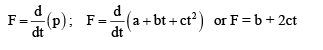Clearly, the force is time-dependent.

Test: Centre Of Mass (Competition Level 1)- 2 - Question 24

A bomb of mass 12 kg, initially at rest explodes into two pieces of masses 4 kg and 8 kg. The speed of the 8 kg mass is 6 m/s. The kinetic energy of the 4 kg mass is

Detailed Solution for Test: Centre Of Mass (Competition Level 1)- 2 - Question 24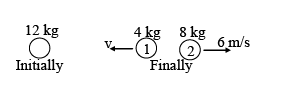Test: Centre Of Mass (Competition Level 1)- 2 - Question 25

A 1 kg ball moving at 12 m/s collides head-on with a 2 kg ball moving in the opposite direction at 24 m/s. If the coefficient of restitution is 2/3 then the final speeds of the two balls are respectively

Detailed Solution for Test: Centre Of Mass (Competition Level 1)- 2 - Question 25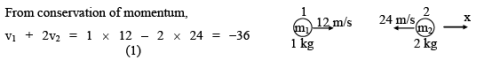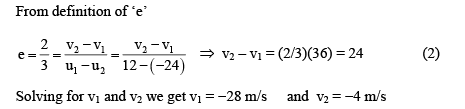Test: Centre Of Mass (Competition Level 1)- 2 - Question 26

Ball 1 collides with another identical ball 2 at rest as shown in figure. For what value of coefficient of restitution e, the velocity of second ball becomes two times that of 1 after collision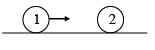Detailed Solution for Test: Centre Of Mass (Competition Level 1)- 2 - Question 26

From conservation of momentum,   mu = mv1 + mv2                      (1)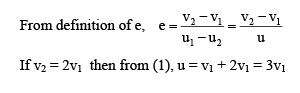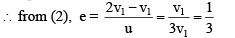Test: Centre Of Mass (Competition Level 1)- 2 - Question 27

“A truck of mass 15 tons moving with one meter per second collides with the stationary truck of 10 tons and they start to move together.
In the above question, the energy lost in the collision is”

Detailed Solution for Test: Centre Of Mass (Competition Level 1)- 2 - Question 27

So, m1v1 = (m1 + m2)v
Hence v = 0.6 m/s
Now, loss in KE = KE initial – KE final = 7500 – 4500 = 3000 J

Test: Centre Of Mass (Competition Level 1)- 2 - Question 28

A particle of mass 1 g moving with a velocity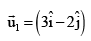m/s experiences a perfectly inelastic collision with another particle of mass 2 g and moving with velocity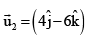m/s. The velocity of the combined particle is

Detailed Solution for Test: Centre Of Mass (Competition Level 1)- 2 - Question 28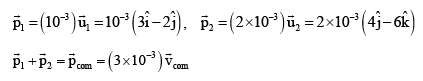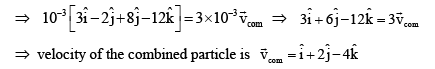Test: Centre Of Mass (Competition Level 1)- 2 - Question 29

A batsman deflects a ball at an angle 90o without changing its initial speed which is equal to 54 km/h. The impulse imparted to the ball whose mass is 0.5 kg is

Detailed Solution for Test: Centre Of Mass (Competition Level 1)- 2 - Question 29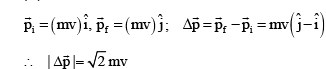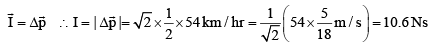Test: Centre Of Mass (Competition Level 1)- 2 - Question 30

A ball moving with a constant speed hits another identical ball at rest. If co-efficient of restitution equals (2/3) then the ratio of speeds of the second ball to that of the first ball after collision will be

Detailed Solution for Test: Centre Of Mass (Competition Level 1)- 2 - Question 30

The final velocities in case of one dimensional semi elastic collision are
V1 = {(m1 - em2)/(m1 + m2)}u1 + {(1 + e)/(m1 + m2)}m2u2
V2 = {(m2 - em1)/(m1 + m2)}u2 + {(1 + e)/(m1 + m2)}m1u1
Here, u1 = u and u2 = 0; e = (2/3) and m1 = m2 = m
By substituting these values we get V2: V1 = 5 : 1

## Physics For JEE

258 videos|633 docs|256 tests
 Use Code STAYHOME200 and get INR 200 additional OFF Use Coupon Code
Information about Test: Centre Of Mass (Competition Level 1)- 2 Page
In this test you can find the Exam questions for Test: Centre Of Mass (Competition Level 1)- 2 solved & explained in the simplest way possible. Besides giving Questions and answers for Test: Centre Of Mass (Competition Level 1)- 2, EduRev gives you an ample number of Online tests for practice

## Physics For JEE

258 videos|633 docs|256 tests